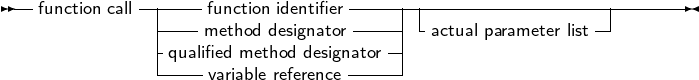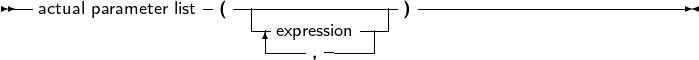### 12.2 Function calls

Function calls are part of expressions (although, using extended syntax, they can be statements too). They are constructed as follows:

_________________________________________________________________________________________________________
Function calls___________________________________________________________________

The  variable reference  must be a procedural type variable reference. A method designator can only be used inside the method of an object. A qualified method designator can be used outside object methods too. The function that will get called is the function with a declared parameter list that matches the actual parameter list. This means that

1. The number of actual parameters must equal the number of declared parameters (unless default parameter values are used).

2. The types of the parameters must be compatible. For variable reference parameters, the parameter types must be exactly the same.

If no matching function is found, then the compiler will generate an error. Which error depends – among other things – on whether the function is overloaded or not: i. e. multiple functions with the same name, but different parameter lists.

There are cases when the compiler will not execute the function call in an expression. This is the case when assigning a value to a procedural type variable, as in the following example in Delphi or Turbo Pascal mode:

```Type
FuncType = Function: Integer;
Var A : Integer;
begin
A := A+1;
end;
Var F : FuncType;
N : Integer;
begin
A := 0;
N := AddOne; { N := 1 !!}
end.
```

In the above listing, the assignment to F will not cause the function AddOne to be called. The assignment to N, however, will call AddOne.

Sometimes, the call is desired, for instance in recursion in that case, the call must be forced. This can be done by adding the parenthesis to the function name:

```function rd : char;

var
c : char;

begin
if (c='\') then
c:=rd();
rd:=c;
end;

var ch : char;

begin
ch:=rd;
writeln(ch);
end.
```

The above will read a character and print it. If the input is a backslash, a second character is read.

A problem with this syntax is the following construction:

```If F = AddOne Then
DoSomethingHorrible;
```

Should the compiler compare the addresses of F and AddOne, or should it call both functions, and compare the result? In fpc and objfpc mode this is solved by considering a procedural variable as equivalent to a pointer. Thus the compiler will give a type mismatch error, since AddOne is considered a call to a function with integer result, and F is a pointer.

How then, should one check whether F points to the function AddOne? To do this, one should use the address operator @:

```If F = @AddOne Then
WriteLn ('Functions are equal');
```

The left hand side of the boolean expression is an address. The right hand side also, and so the compiler compares two addresses. How to compare the values that both functions return ? By adding an empty parameter list:

```  If F()=Addone then
WriteLn ('Functions return same values ');
```

Remark that this last behavior is not compatible with Delphi syntax. Switching on Delphi mode will allow you to use Delphi syntax.